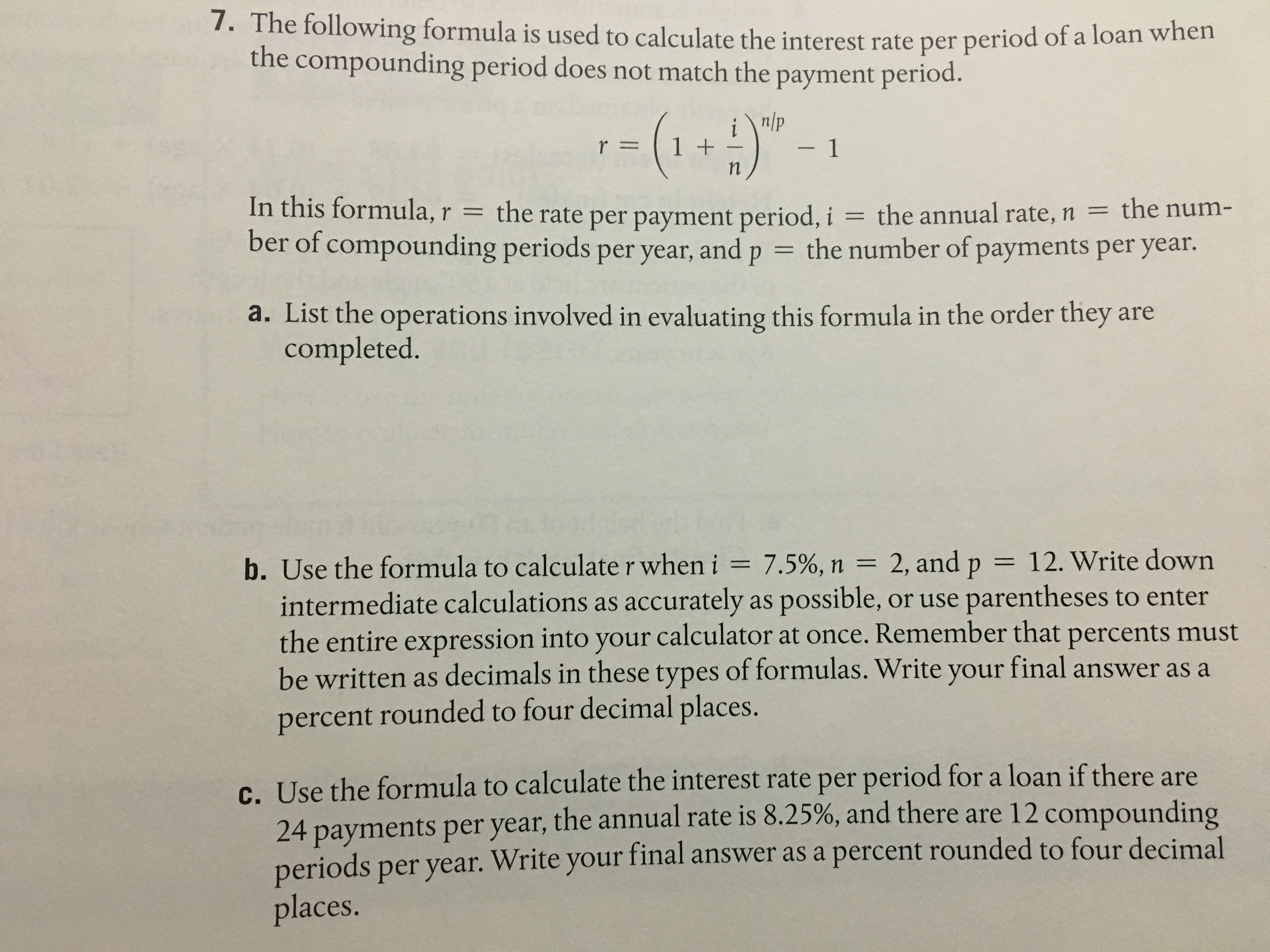# 7. The following formula is used to calculate the interest rate per period o f a loan when the compounding period does not match the payment period. n/p r 11 In this formula,r the rate per payment period, i the annual rate, n the num- ber of compounding periods per year, and p the number of payments per year. a. List the operations involved in evaluating this formula in the order they are completed. b. Use the formula to calculate r when i 7.5%, n 2, and p 12. Write down intermediate calculations as accurately as possible, or use parentheses to enter the entire expression into your calculator at once. Remember that percents must be written as decimals in these types of formulas. Write your final answer as a percent rounded to four decimal places. c. Use the formula to calculate the interest rate per period for a loan if there are 4 payments per year, the annual rate is 8.25%, and there are 12 compounding periods per year. Write your final answer as a places

Questionhelp_outlineImage Transcriptionclose7. The following formula is used to calculate the interest rate per period o f a loan when the compounding period does not match the payment period. n/p r 11 In this formula,r the rate per payment period, i the annual rate, n the num- ber of compounding periods per year, and p the number of payments per year. a. List the operations involved in evaluating this formula in the order they are completed. b. Use the formula to calculate r when i 7.5%, n 2, and p 12. Write down intermediate calculations as accurately as possible, or use parentheses to enter the entire expression into your calculator at once. Remember that percents must be written as decimals in these types of formulas. Write your final answer as a percent rounded to four decimal places. c. Use the formula to calculate the interest rate per period for a loan if there are 4 payments per year, the annual rate is 8.25%, and there are 12 compounding periods per year. Write your final answer as a places fullscreen

### Want to see the step-by-step answer?